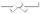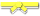# Exercises

0 / 10

Given a binary tree, write a recursive function to return the difference between the sum of all node values at odd levels and sum of all...0 / 10

The height of a binary tree is the length of the path to the deepest node. An empty tree has a height of 0, a tree with one node has a...0 / 10

Write a recursive function that increments by one the value for every node in the binary tree pointed at by `root`, then returns the...0 / 10

Write a recursive function to count the number of leaf nodes in the binary tree pointed at by `root`. You must use the `isLeaf` method to...0 / 10

We define a "root-to-leaf path" to be any sequence of nodes in a tree starting with the root node and proceeding downward to a leaf. The...0 / 10

Write a recursive function to set the value for each node in a binary tree to be its depth then return the modified tree. Assume that...0 / 10

Write a recursive function that returns the sum of the values for all of the nodes of the binary tree with root `root`.
Here are methods...0 / 10

Given two binary trees, return true if and only if they are mirror images of each other. Note that two empty trees are considered mirror...0 / 10

Given two binary trees, return true if they are identical (they have nodes with the same values, arranged in the same way).
Here are...0 / 10

Given two binary trees, return true if and only if they are structurally identical (they have the same shape, but their nodes can have...0 / 10

Consider the following class definition:
Object data;
}
Create a new node with info 'D' and insert it at the end...0 / 10

Consider the following class definition:
Object data;
}
Write a while loop to make q refer successively to each...0 / 10

Consider the following class definition:
Object data;
}0 / 10

Consider the following class definition:

``class Link{ public Object data; public Link next; } ``

In the method below, you will receive a...0 / 10

Consider the following class definition:
Object data;
}
Return a reference to the second to last node (null if...0 / 50

Write a member method balanceBags in Java that implements the following logic: Given a String parameter the methods returns the String...0 / 10

Consider the following class definition:
Object data;
}
List L has a reference p to the first node. Write code...0 / 10

Consider the following class definition:
Object data;
}
List L has a reference p to the first node. Write code...0 / 10

Consider the following class definition:
Object data;
}
List L has a reference p to the first node. Write code...0 / 10

Consider the following class definition:
Object data;
}
List L has a reference p to the first node. Write code...0 / 10

Consider the following class definition:
Object data;
}
p is a reference to the first node in a List L. Create a...0 / 50

Write the member method 'playBagBingo()' that implements the player logic for the game described below. Bag Bingo is a game of chance...0 / 30

For this assignment, create a method that will turn a fixed-size arrayBag into a set.

A Set is an abstract data type somewhat similar...0 / 10

Consider the following class definition:Write an `ArrayBasedStack<T>` member method called `reverseString()` that takes a String as a parameter, then uses an ArrayBasedStack...